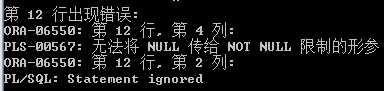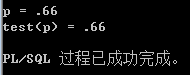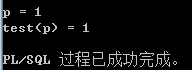# PLSQL Language Reference-PL/SQL子程序-子程序参数-形参和实参

## 子程序参数

### 形参和实参

 DECLARE     emp_num NUMBER(6) := 120;     bonus   NUMBER(6) := 100;     merit   NUMBER(4) := 50;       PROCEDURE raise_salary (         emp_id NUMBER,  -- 形参         amount NUMBER   -- 形参     ) IS     BEGIN         UPDATE employees         SET salary = salary + amount  -- 引用形参         WHERE employee_id = emp_id;   -- 引用形参     END raise_salary;   BEGIN   raise_salary(emp_num, bonus);          -- 实参   raise_salary(emp_num, merit + bonus);  -- 实参  END; /

1）约束子类型的形参

n  如果子类型有NOT NULL约束，则实参继承它

n  如果子类型的基类型为VARCHAR2，则实参不继承子类型的size

n  如果子类型的基类型为数值类型，则实参继承它的range，但不继承它的precisionscale

 DECLARE     SUBTYPE License IS VARCHAR2(7) NOT NULL;     n  License := 'DLLLDDD';       PROCEDURE p (x License) IS     BEGIN         DBMS_OUTPUT.PUT_LINE(x);     END;   BEGIN     p('1ABC123456789');  -- 成功,因为大小没有继承来     p(NULL);               -- 产生错误, NOT NULL被继承来了 END;--只继承范围，不继承精度 DECLARE     FUNCTION test (p INTEGER) RETURN INTEGER IS     BEGIN         DBMS_OUTPUT.PUT_LINE('p = ' || p);         RETURN p;     END test;   BEGIN     DBMS_OUTPUT.PUT_LINE('test(p) = ' || test(0.66)); END;DECLARE     FUNCTION test (p NUMBER) RETURN NUMBER IS         q INTEGER := p;  -- 隐式将p转换为INTEGER     BEGIN         DBMS_OUTPUT.PUT_LINE('p = ' || q);  -- 显示q，而不是p         RETURN q;                           -- 返回q，而不是p     END test;   BEGIN     DBMS_OUTPUT.PUT_LINE('test(p) = ' || test(0.66)); END;• 博文量
1986
• 访问量
5569016